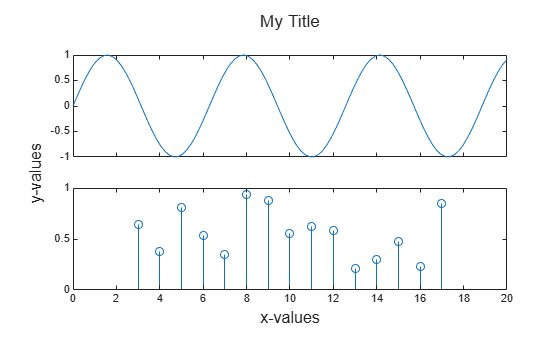# Combine Multiple Plots

This example shows how to combine plots in the same axes using the `hold` function, and how to create multiple axes in a figure using the `tiledlayout` function. The `tiledlayout` function is available starting in R2019b. If you are using an earlier release, use the `subplot` function instead.

### Combine Plots in Same Axes

By default, new plots clear existing plots and reset axes properties, such as the title. However, you can use the `hold on` command to combine multiple plots in the same axes. For example, plot two lines and a scatter plot. Then reset the hold state to off.

```x = linspace(0,10,50); y1 = sin(x); plot(x,y1) title('Combine Plots') hold on y2 = sin(x/2); plot(x,y2) y3 = 2*sin(x); scatter(x,y3) hold off```When the hold state is on, new plots do not clear existing plots or reset axes properties, such as the title or axis labels. The plots cycle through colors and line styles based on the `ColorOrder` and `LineStyleOrder` properties of the axes. The axes limits and tick values might adjust to accommodate new data.

### Display Multiple Axes in a Figure

You can display multiple axes in a single figure by using the `tiledlayout` function. This function creates a tiled chart layout containing an invisible grid of tiles over the entire figure. Each tile can contain an axes for displaying a plot. After creating a layout, call the `nexttile` function to place an axes object into the layout. Then call a plotting function to plot into the axes. For example, create two plots in a 2-by-1 layout. Add a title to each plot.

Note: This code uses the `tiledlayout` function, which is available starting in R2019b. If you are using an earlier release, use the `subplot` function instead.

```x = linspace(0,10,50); y1 = sin(x); y2 = rand(50,1); tiledlayout(2,1) % Requires R2019b or later % Top plot nexttile plot(x,y1) title('Plot 1') % Bottom plot nexttile scatter(x,y2) title('Plot 2')```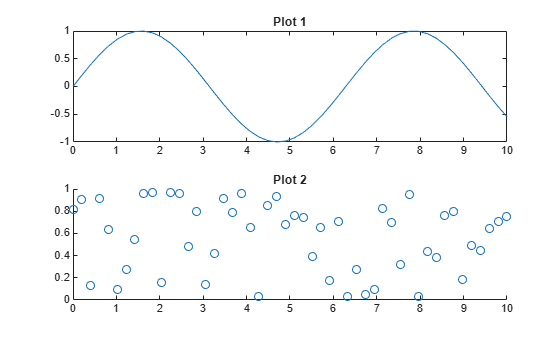### Create Plot Spanning Multiple Rows or Columns

To create a plot that spans multiple rows or columns, specify the `span` argument when you call `nexttile`. For example, create a 2-by-2 layout. Plot into the first two tiles. Then create a plot that spans one row and two columns.

```x = linspace(0,10,50); y1 = sin(x); y2 = rand(50,1); % Top two plots tiledlayout(2,2) % Requires R2019b or later nexttile plot(x,y1) nexttile scatter(x,y2) % Plot that spans nexttile([1 2]) y2 = rand(50,1); plot(x,y2)```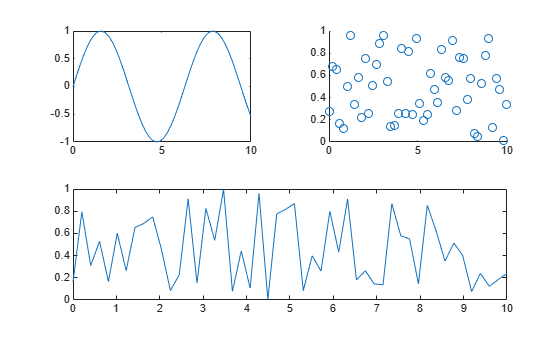### Modify Axes Appearance

Modify the axes appearance by setting properties on each of the axes objects. You can get the axes object by calling the `nexttile` function with an output argument. You also can specify the axes object as the first input argument to a graphics function to ensure that the function targets the correct axes.

For example, create two plots and assign the axes objects to the variables `ax1` and `ax2`. Change the axes font size and x-axis color for the first plot. Add grid lines to the second plot.

```x = linspace(0,10,50); y1 = sin(x); y2 = rand(50,1); tiledlayout(2,1) % Requires R2019b or later % Top plot ax1 = nexttile; plot(ax1,x,y1) title(ax1,'Plot 1') ax1.FontSize = 14; ax1.XColor = 'red'; % Bottom plot ax2 = nexttile; scatter(ax2,x,y2) title(ax2,'Plot 2') grid(ax2,'on')```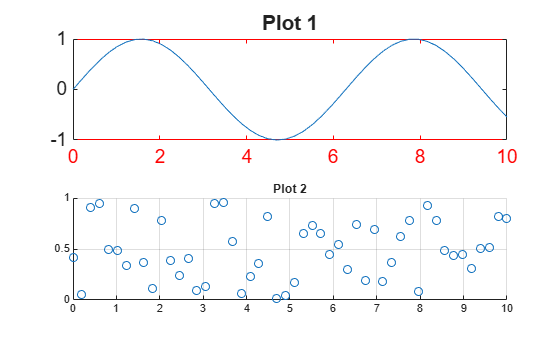### Control Spacing Around the Tiles

You can control the spacing around the tiles in a layout by specifying the `Padding` and `TileSpacing` properties. For example, display four plots in a 2-by-2 layout.

```x = linspace(0,30); y1 = sin(x); y2 = sin(x/2); y3 = sin(x/3); y4 = sin(x/4); % Create plots t = tiledlayout(2,2); % Requires R2019b or later nexttile plot(x,y1) nexttile plot(x,y2) nexttile plot(x,y3) nexttile plot(x,y4)```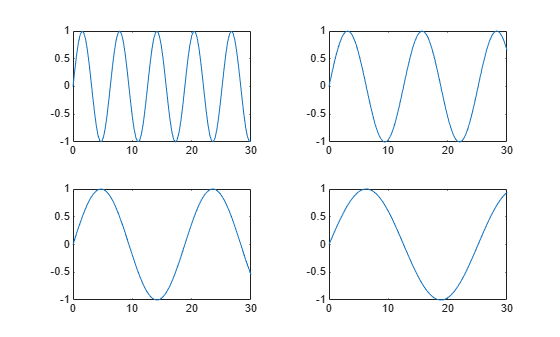Reduce the spacing around the perimeter of the layout and around each tile by setting the `Padding` and `TileSpacing` properties to `'compact'`.

```t.Padding = 'compact'; t.TileSpacing = 'compact';```### Display Shared Title and Axis Labels

You can display a shared title and shared axis labels in a layout. Create a 2-by-1 layout `t`. Then display a line plot and a stem plot. Synchronize the x-axis limits by calling the `linkaxes` function.

```x1 = linspace(0,20,100); y1 = sin(x1); x2 = 3:17; y2 = rand(1,15); % Create plots. t = tiledlayout(2,1); % Requires R2019b or later ax1 = nexttile; plot(ax1,x1,y1) ax2 = nexttile; stem(ax2,x2,y2) % Link the axes linkaxes([ax1,ax2],'x');```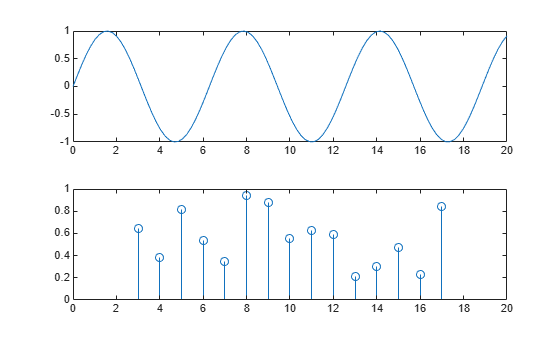Add a shared title and shared axis labels by passing `t` to the `title`, `xlabel`, and `ylabel` functions. Move the plots closer together by removing the x-axis tick labels from the top plot and setting the `TileSpacing` property of `t` to `'compact'`.

```% Add shared title and axis labels title(t,'My Title') xlabel(t,'x-values') ylabel(t,'y-values') % Move plots closer together xticklabels(ax1,{}) t.TileSpacing = 'compact';```